# Current Density¶

Current densityis a vector field in the same direction as current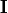but with magnitude of current per area. It may also be represented as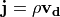, where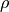is charge density (i.e. charge per volume) andis the drift velocity of charged particles. Current density is related to electric field via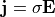via the conductivity constant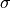of the material. See Wikipedia:Current_density for background.

## SIMION specific¶

SIMION in its poisson solver (SIMION 8.1) can solve for current density throughout space when given a conductivity () throughout space and boundary conditions on electric potential. The governing Poisson Equation is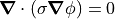which is the same as equation used in dielectrics problems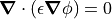but with the dielectric constantreplaced with.

SIMION 8.2 can also utilize a known distribution of current density for calculating (vector) Magnetic Potential and hence magnetic fields. (See Magnetic Potential for details.)

Note

This page is abridged from the full SIMION "Supplemental Documentation" (Help file). The following additional sections can be found in the full version of this page accessible via the "Help > Supplemental Documentation" menu in SIMION 8.1.1 or above:
• SIMION Modeling Details - Calculating current density from conductivity
• Plotting current density vector fields